## Producing Waveforms using Wave Generator module in the PSLab Android App

This blog will demonstrate how to produce different waveforms using the Wave Generator module in the PSLab android app and view them on the Oscilloscope.

The Wave Generator in PSLab android app is simple to use and has a UI which is similar to physical commodity wave generators. It is capable of producing different waveforms like sine, sawtooth and square wave.

## Apparatus Required

Before getting started with the wave generator we require the following items:

1. PSLab device
2. An android phone with PSLab app installed in it.
3. USB cable (Mini B)
4. OTG(On the Go) wire
5. Some connecting wires having pins at both ends

## Understanding the Wave Generator Pins

Let me briefly explain the use of the pins that are going to be used in the Wave generator module:

#### S1 and S2 pins

The PSLab device contains two pins (S1, S2) which are capable of producing two independent analog waveforms (sine,  sawtooth) having different frequencies and phase offset. The frequency range is from 10Hz to 5Khz.

#### SQR1, SQR2, SQR3 and SQR4 pin

The SQR1 pin is used for producing the square waveform and all the SQ pins can be used together to produce four different PWM signal having the same frequency. These PWM signal can have a different duty cycle and phase.

#### CH1, CH2 and CH3 pin

The CH pins are used by the oscilloscope in the  PSLab android app to monitor waveform signals produced by the wave generator pins. They can be used together to simultaneously monitor multiple waveforms.

## Setting up the Device

We need to connect the PSLab device with the mobile phone as shown in Figure 2 which can be done by following steps:

1. Connect a micro USB(Mini B) to the PSLab device.
2. Connect the other end of the micro USB cable to the OTG.
3. Connect the OTG to the phone.

## Producing Waveforms

Now, once the device has been properly connected to the device (which is shown at the top right corner of the app), then in the instruments page scroll down to the Wave Generator card and click on it to open the WaveGenerator activity.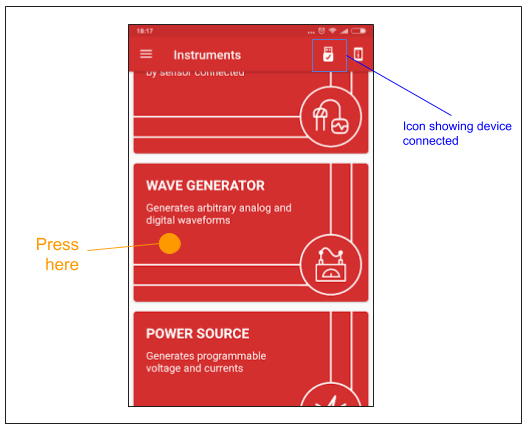Figure 3 shows the instruments containing card view to all the instruments and icon to show device status

Here you will see a screen like shown in Figure 4 containing two monitors and a controlling panel with lots of buttons. Here the Waveform panel is used to control the S1 and S2 pins whose properties are shown on the left monitor screen and the Digital panel is used to control the SQR pins whose properties are shown on the right monitor screen.

### For sine/sawtooth wave:

Connect the S1 pin to the CH1 pin using a connecting wire, then in the Waveform panel select the Wave1 button, choose the type of waveform(either sine or sawtooth), then click on the Freq button to change the frequency of the wave, then use the Seek bar or the up/down arrow buttons to change the value of frequency and then press the set button to set the frequency for the S1 pin as shown below:Figure 5 The GIF shows the setting of the properties of the W1 pin in the UI

Now, click the view button at bottom right corner, this will directly open the Oscilloscope provided by the PSLab android app .

Once the oscilloscope is open, check the CH1 pin from the panel in the bottom and we can see the sine wave in the monitor shown by the screen in Figure 6 and Figure 7

Similarly, if you want to see two sine waves connect the S1 pin to the CH1 and connect the S2 pin to the CH2 channel , choose the wave-type for both pin, set the frequencies for both of the waves, here you can also set the phase difference between the two waves, for setting phase difference first click on Wave2 button it will enable the phase button, then click on the Phase button and set the value of phase with the help of the Seek bar.

### For Square Wave

Connect the CH1 pin to the SQ1 pin, after making the connection head over to the Digital panel in the Wave Generator, ensure that the mode is selected to square, now click on the Freq button in the digital panel and set the frequency of the square wave with the help of Seek bar, then click on the Duty button and set the value of duty cycle for the square wave as shown below: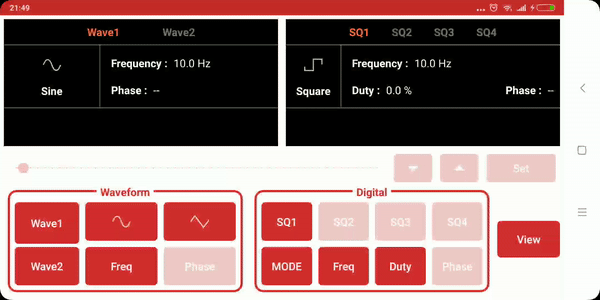Figure 8 The GIF shows the setting of properties for producing square wave from SQ1 pin

Now, once the square wave has been set click on the view button, the oscilloscope will open then select the CH1 pin and you can see the square wave on the monitor as shown by the screen in Figure 9.

Thus we have produced different waveforms using PSLab wave generator module.

## Resources

PSLab device pin diagram  – https://github.com/fossasia/pslab-artwork/blob/master/Sticker/pslabdesign.png

Continue Reading Producing Waveforms using Wave Generator module in the PSLab Android App

## Electrical Experiments with PSLab

PSLab has the capability to perform a variety of experiments. The PSLab Android App and the PSLab Desktop App have built-in support for over 70 experiments which are commonly performed by students. In addition to that, it can be used in other experiments conveniently. This blog post is in continuation with the previous two posts regarding performing experiments (links in the reference) and this blog deals with another category of experiments that can be performed using PSLab.

The blog lists experiments which mainly involve the basic circuit elements like resistors, capacitors and inductors. These experiments involve the study of R-C, L-R, L-C and L-C-R circuits. These circuits have properties which make them important in real life applications and this blog attempts to give a rough picture of their importance.

Ohm’s Law, Capacitive Reactance and Inductive Reactance

These experiments involve the study of each of the basic circuit element individually. The current and voltage characteristics of each of the elements is studied.

The definitions of the above are:

Ohm’s Law – This is a law familiar to most. It relates the voltage and current of a purely resistive circuit stating that the voltage and current are proportional to each other and their ratio is a constant called the resistance. In this case, the current and voltage are in the same phase.

Capacitive Reactance – Across a capacitor in an AC circuit, the current and voltage are not in the same phase and the current leads the voltage. For a purely capacitive circuit, this difference is 90o.

Inductive Reactance –  Across an inductor in an AC circuit, the current and voltage are not in the same phase and the current lags behind the voltage. For a purely inductive circuit, this difference is 90o.

The reactance is given for capacitor and inductor is given by 1/wC and wL respectively, where C & L are the values of capacitance and inductance respectively and w is the frequency of the AC signal.

The circuit for the setup is shown below. We need to observe the plot of the input waveform and the plot of the voltage across individual elements to observe the phase shift.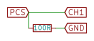1. Connect CH1 & GND across the input terminals and CH2 & GND across the terminals of any of the elements.
2. An external signal can be used or can be generated using the PSLab. Use the PSLab to generate a sinusoidal signal of frequency 1000 Hz. by connecting the ends of PV1 in the circuit.
3. Observe the waveforms. In case of the resistor, there should be no observable phase lag between the two. In case of the capacitor and inductor, there will be an observable phase difference of 90o.
4. For the capacitive and inductive circuits, just replace the resistor in the above circuit with capacitor/inductor.

RC Circuits

Drawing their names from their respective calculus functions, the integrator produces a voltage output proportional to the product (multiplication) of the input voltage and time; and the differentiator (not to be confused with differential) produces a voltage output proportional to the input voltage’s rate of change.

RC Integrator circuit

For constructing the RC integrator circuit, connect the circuit as shown in the diagram.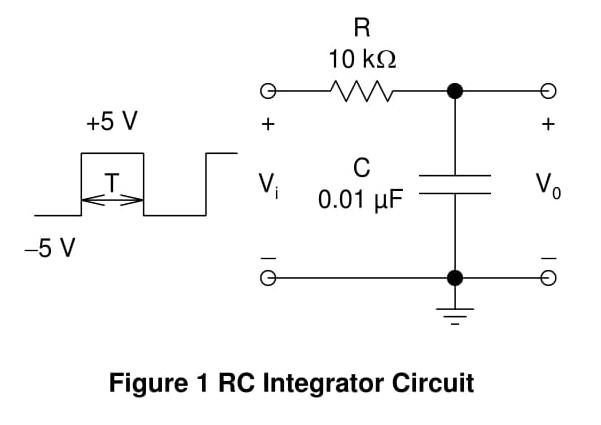• Construction of the integrator circuit is fairly simple once the differentiator circuit is done.
• Interchange the positions of the capacitor and resistor in the above circuit and the circuit for the integrator is complete.
• Observe the output waveform. Plot both the CH1 and CH2 data simultaneously to compare the waveforms.

RC Differentiator circuit

For constructing the RC differentiator circuit, connect the circuit as shown in the diagram.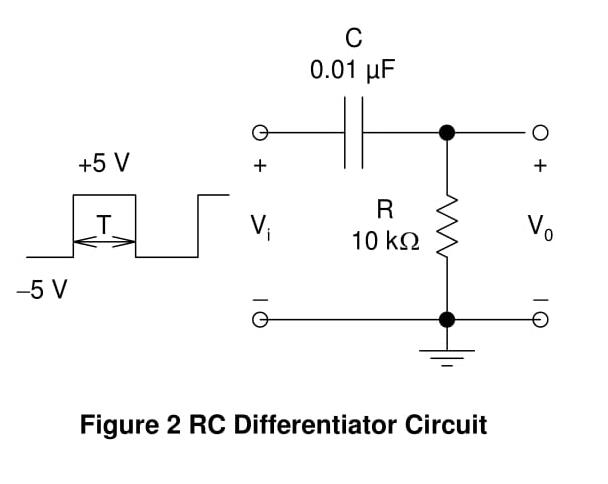• The values of resistance and capacitance used here are 10k ohm and 0.01uF.
• Connect the CH1 and GND pins of the board with the input side marked as Vi. Ensure that GND is connected to the GND of the circuit.
• Similarly, connect CH2 and GND with the corresponding ends of the output side marked as Vo.
• PSLab can also be used for supplying the input to the circuit. Connect the ends of W1 and GND across Vi. W1 can be used to generate a square wave of 10V peak to peak voltage with a frequency of 500 Hz.
• Observe the output waveform. Plot both the CH1 and CH2 data simultaneously to compare the waveforms.

RL Circuits

RL Integrator Circuit.

For constructing the RL integrator circuit, connect the circuit as shown in the diagram.• Construction of the integrator circuit is fairly simple once the differentiator circuit is done.
• Interchange the positions of the inductor and resistor in the above circuit and the circuit for the integrator is complete.
• Observe the output waveform. Plot both the CH1 and CH2 data simultaneously to compare the waveforms.

RL Differentiator Circuit

For constructing the RL differentiator circuit, connect the circuit as shown in the diagram.• The values of resistance and inductance used here are 470 ohm and 10mH.
• Connect the CH1 and GND pins of the board with the input side marked as Vi. Ensure that GND is connected to the GND of the circuit.
• Similarly, connect CH2 and GND with the corresponding ends of the output side marked as Vo.
• PSLab can also be used for supplying the input to the circuit. Connect the ends of W1 and GND across Vi. W1 can be used to generate a square wave of 2V peak to peak voltage with a frequency of 5000 Hz.
• Observe the output waveform. Plot both the CH1 and CH2 data simultaneously to compare the waveforms.

Frequency Response

Frequency Response of an electric or electronics circuit allows us to see exactly how the output gain (known as the magnitude response) and the phase (known as the phase response) changes at a particular single frequency, or over a whole range of different frequencies from 0Hz, (d.c.) to many thousands of megahertz, (MHz) depending upon the design characteristics of the circuit.

Frequency response of a circuit can be studied using different tools like Bode plots, phase plots etc. However, this blog would limit to using simple RC and RL circuits as they can be easily visualised using an oscilloscope.

RC Circuits

• For observing the frequency response of RC circuits, the circuit can be constructed as shown below.
• The values of resistance and capacitance used here are 10k ohm and 0.01uF.
• Connect the CH1 and GND pins of the board with the input side marked as Vi. Ensure that GND is connected to the GND of the circuit.
• Similarly, connect CH2 and GND with the corresponding ends of the output side marked as Vo.
• PSLab can also be used for supplying the input to the circuit. Connect the ends of W1 and GND across Vi. W1 can be used to generate a square wave of 10V peak to peak voltage with a frequencies ranging from 100 Hz to 5000 Hz.
• Switch to the X-Y mode of the oscilloscope and observe the waveform formed.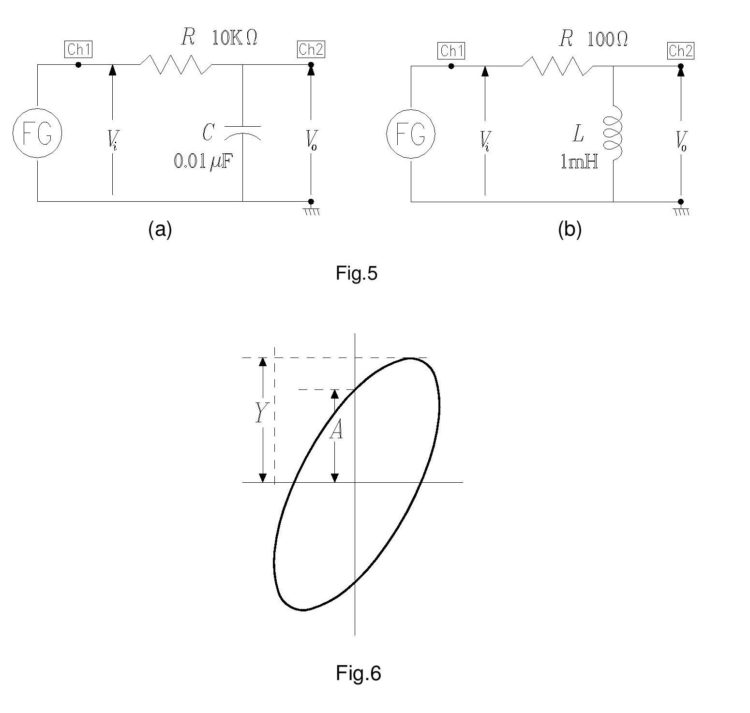RL Circuits

• For observing the frequency response of RL circuits, the circuit can be constructed as shown below.
• The values of resistance and inductance used here are 470 ohm and 10mH.
• Connect the CH1 and GND pins of the board with the input side marked as Vi. Ensure that GND is connected to the GND of the circuit.
• Similarly, connect CH2 and GND with the corresponding ends of the output side marked as Vo.
• Note: PSLab in this case cannot be used as an AC source as the maximum frequency of waveforms produced by PSLab is limited to 5kHz. However, this experiment would also need frequencies much higher than 5 Hz i.e upto 50 kHz. So, a dedicated function generator is needed. However, the oscilloscope would work just fine.
• Switch to the X-Y mode of the oscilloscope and observe the waveform formed.

References:

1. The previous blog on experiments using PSLab focusing in electronics https://blog.fossasia.org/electronics-experiments-with-pslab/
2. The previous blog on experiments using PSLab involving some general experiments https://blog.fossasia.org/fascinating-experiments-with-pslab/
Continue Reading Electrical Experiments with PSLab

## Fascinating Experiments with PSLab

PSLab can be extensively used in a variety of experiments ranging from the traditional electrical and electronics experiments to a number of innovative experiments. The PSLab desktop app and the Android app have all the essential features that are needed to perform the experiments. In addition to that there is a large collection of built-in experiments in both these experiments.

This blog is an extension to the blog post mentioned here. This blog lists some of the basic electrical and electronics experiments which are based on the same principles which are mentioned in the previous blog. In addition to that, some interesting and innovative experiments where PSLab can be used are also listed here. The experiments mentioned here require some prerequisite knowledge of electronic elements and basic circuit building. (The links mentioned at the end of the blog will be helpful in this case)

Op-Amp as an Inverting and a Non-Inverting AmplifierThere are two methods of doing this experiment. PSLab already has a built-in experiment dedicated to inverting and non-inverting amplification of op-amps. In the Android App, just navigate to Saved Experiments -> Electronics Experiments -> Op-Amp Circuits -> Inverting/ Non-Inverting. In case of the Desktop app, select Electronics Experiments from the main drop-down at the top of the window and select the Inverting/Non-inverting op-amp experiment.

This experiment can also performed using the basic features of PSLab. The only advantage of this methodology is that it allows much more tweaking of values to observe the Op-Amp behaviour in greater detail. However, the built-in experiment is good enough for most of the cases.

• Construct the above circuits on a breadboard.
• For the amplifier, connect the terminals of CH1 and GND of PSLab on the input side i.e. next to Vi and the terminals of CH2 and GND on the output side i.e next to Vo.
• Usually, an Op-Amp like LM741 have a set of pins, one dedicated for the inverting input and the other dedicated for the non-inverting input. It is recommended to consult the datasheet of the Op-Amp IC used in order to get the pin number with which the input has to be connected.
• The terminals of W1 and GND are also connected on the input side and they are used to generate a sine wave.
• The resistors displayed in the figure have the values R1 = 10k and R2 = 51k. Resistance values other than these can also be considered. The gain of the op-amp would depend on the ratio of R2/R1, so it is better to consider values of R2 which are significantly larger than R1 in order to see the gain properly.
• Use the PSLab Desktop App and open the Waveform Generator in Control. Set the wave type of W1 to Sine and set the frequency at 1 kHz and magnitude to 0.1 V. Then go ahead and open the Oscilloscope.
• CH1 would display the input waveform and CH2 will display the output waveform and the plots can be observed.
• If the input is connected to the inverting pin of the op-amp, the output obtained will be amplified and will have a phase difference of 90o with the input waveform whereas when the non-inverting pin is selected, the output is just amplified and no such phase difference is observed.
• Note: Take proper care while connecting the V+ and V- pins of the op-amp, else the op-amp will be damaged.

Diode as an Integrator and Differentiator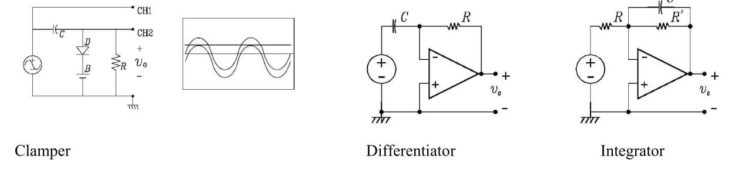An integrator in measurement and control applications is an element whose output signal is the time integral of its input signal. It accumulates the input quantity over a defined time to produce a representative output.

Integration is an important part of many engineering and scientific applications. Mechanical integrators are the oldest application, and are still used in such as metering of water flow or electric power. Electronic analogue integrators are the basis of analog computers and charge amplifiers. Integration is also performed by digital computing algorithms.

In electronics, a differentiator is a circuit that is designed such that the output of the circuit is approximately directly proportional to the rate of change (the time derivative) of the input. An active differentiator includes some form of amplifier. A passive differentiator circuit is made of only resistors and capacitors.

• Construct the above circuits on a breadboard.
• For both the circuits, connect the terminals of CH1 and GND of PSLab on the input side i.e. next to input voltage source and the terminals of CH2 and GND on the output side i.e next to Vo.
• Ensure that the inverting and the non-inverting terminals of the op-amp are connected correctly. Check for the +/- signs in the diagram. ‘+’ corresponds to non-inverting and ‘-’ corresponds to inverting.
• The terminals of W1 and GND are also connected on the input side and they are used to generate a sine wave.
• The resistors displayed in the figure have the values R1 = 10k and R2 = 51k. Resistance values other than these can also be considered. The gain of the op-amp would depend on the ratio of R2/R1, so it is better to consider values of R2 which are significantly larger than R1 in order to see the gain properly.
• Use the PSLab Desktop App and open the Waveform Generator in Control. Set the wave type of W1 to Sine and set the frequency at 1 kHz and magnitude to 5V (10V peak to peak). Then go ahead and open the Oscilloscope.
• CH1 would display the input waveform and CH2 will display the output waveform and the plots can be observed.
• If all the connections are made properly and the values of the parameters are set properly, then the waveform obtained should be as shown below.

Performing experiments involving ICs (Digital circuits)

The experiments mentioned so far including the ones mentioned in the previous blog post involved analog circuits and so they required features like the arbitrary waveform generator. However, digital circuits work using discrete values only. PSLab has the features needed to perform digital experiments which mainly involve the use of a square wave generator with a variable duty cycle.

PSLab board has dedicated pins named SQR1, SQR2, SQR3 and SQR4. The options for configuring these pins is present under the Advanced Control section in the Desktop app and in the Android app Applications->Control->Advanced. The options include selecting the pins which we want to use for digital outputs and then configuring the frequency and duty cycle of the square wave generated from that particular pin.

Innovative Experiments using PSLab

PSLab has quite a good number of interesting built-in experiments. These experiments can be found in the dropdown list at the top in the Desktop App and under the Saved Experiments header in the Android App. The built-in experiments come bundled with good quality documentation having circuit diagrams and detailed procedure to perform the experiments.

Some of the interesting experiments include:

• Lemon Cell Experiment: In this experiment, the internal resistance and the voltage supplied by the lemon cell are measured.• Sound Beats: In acoustics, a beat is an interference pattern between two sounds of slightly different frequencies, perceived as a periodic variation in volume whose rate is the difference of the two frequencies.• When tuning instruments that can produce sustained tones, beats can readily be recognized. Tuning two tones to a unison will present a peculiar effect: when the two tones are close in pitch but not identical, the difference in frequency generates the beating.
• This experiment requires producing two waves together of different frequencies and connecting them to the same oscilloscope channel. The pattern observed is shown below.

References:

1. The previous blog on experiments using PSLab – https://blog.fossasia.org/electronics-experiments-with-pslab/
2. More about op-amps and their characteristics – http://www.electronics-tutorials.ws/opamp/opamp_1.html
4. Experiments involving digital circuits for reference – http://web.iitd.ac.in/~shouri/eep201/experiments.php
Continue Reading Fascinating Experiments with PSLab

## Electronics Experiments with PSLab

Numerous college level electronics experiments can be performed using Pocket Science Lab (PSLab). The Android app and the Desktop app have all the essential features needed to perform these experiments and both these apps have quite a large number of experiments built-in. Some of the common experiments involve the use of BJT (Bipolar Junction Transistor), Zener Diode, FET (Field Effect Transistor), Op-Amp ( Operational Amplifier) etc. This blog walks through the details of performing some experiments using the above commonly used elements.

The materials required for all the experiments are minimal and includes a few things like PSLab hardware device, components like Diodes, Transistors, Op-Amps etc., connecting wires/jumpers and secondary components like resistors, capacitors etc. Most of these elements would be a part of the PSLab Accessory Kit.

It is recommended to read this blog here, go through the resources mentioned at the end and also get acquainted with construction of circuits before advancing with the experiments mentioned in this blog.

Half Wave and Full Wave Rectifiers

The Bipolar Junction Transistor (BJT) can be used as a rectifier. Rectifiers are needed in circuits to obtain a nearly constant and stable output voltage and prevent any ripples in the circuit. The rectifier can be half wave or full wave depending on whether it rectifies one or both cycles of Alternating Voltage.

The circuit for the Half and Full Wave rectifier is given as follows:• Construct the above circuits on a breadboard.
• For the half wave rectifier, connect the terminals of CH1 and GND of PSLab on the input side and the terminals of CH2 and GND on the output side.
• The terminals of W1 and GND are also connected on the input side and they are used to generate a sine wave.
• Use the PSLab Desktop App and open the Waveform Generator in Control. Set the wave type of W1 to Sine and set the frequency at 100 Hz and magnitude to 10mV. Then go ahead and open the Oscilloscope.
• CH1 would display the input waveform and CH2 will display the output waveform and the plots can be observed.
• The plot obtained will have rectification in only half of the cycle. In order to obtain rectification in the complete cycle, the full wave rectifier is needed.
• For the full wave rectifier, the procedure is the same but an additional diode is used. Use an additional channel CH3 to plot the extra input.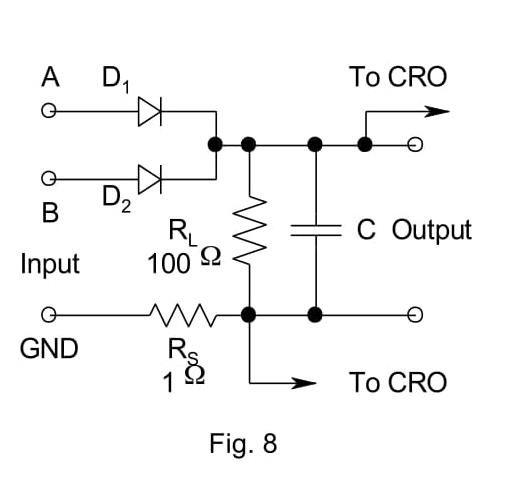• The plot obtained from the above steps would still have ripples and so a capacitor is placed in parallel to cancel this effect.
• Place a 100uF/330uF capacitor in parallel to the resistor RL and an additional 1 ohm resistor in the circuit.

BJT Inverter

• Transistor has a lot of functions. The most common of them is its use as an amplifier. However, transistor can be used as a switch in a circuit i.e. as an inverter.
• The circuit for this experiment is shown below. For this experiment, it is recommended to use an external 5V DC supply like a battery. Connect the transistor and the diode initially and then connect the resistors accordingly. (Connect the terminals of diode and transistor carefully else they will be damaged).
• When the circuit is constructed completely, connect CH1 to Vi and CH2 to Vo. Vi and Vo are input and outputs respectively and are marked in the figure.
• The terminals of W1 and GND are also connected on the input side and they are used to generate a sine wave.
• Use the PSLab Desktop App and open the Waveform Generator in Control. Set the wave type of W1 to Sine and set the frequency at 200 Hz and magnitude to 10mV.
• Then go ahead and open the Oscilloscope. Use the X-Y mode of the oscilloscope to obtain the plot between Vi and Vo which should look like the graph shown below.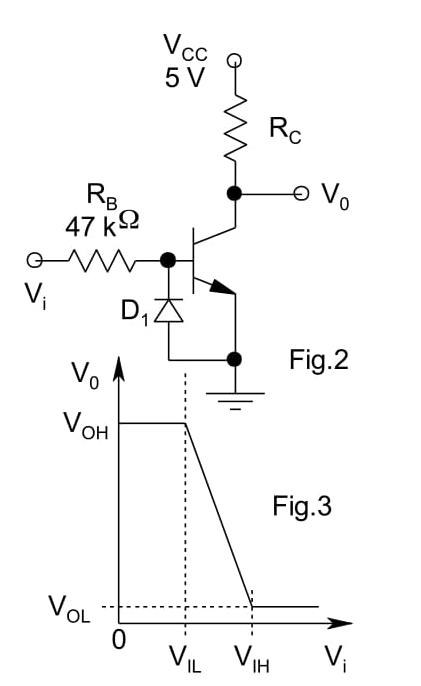Common Mode Gain and Differential Mode Gain in Op-Amps

Gain of any amplifier can be calculated by calculating the ratio of the output and input voltage. On plotting the graph in X-Y mode, a Vo vs Vi graph is obtained. The slope of that graph gives us the gain at any particular input voltage.

• For finding the Differential Mode gain of an Op-Amp, construct the circuit as shown below.
• When the circuit is constructed completely, connect CH1 to Vi and CH2 to Vo. Vi and Vo are input and outputs respectively and are marked in the figure. The terminals of W1 and GND are also connected on the input side and they are used to generate a sine wave.
• The power supply provided to the Op-Amp are set to + 12V. (If faced with any confusion, please refer to the resources mentioned at the end of the blog to learn more about Op-Amps before proceeding ahead.)
• Use the PSLab Desktop App and open the Waveform Generator in Control. Set the wave type of W1 to Sine and set the frequency at 1000 Hz and magnitude to 0.5V. Then go ahead and open the Oscilloscope. Use the X-Y mode of the oscilloscope to obtain the plot between Vi and Vo.
• For finding the Common Mode gain of the Op-Amp, remove the waveform generator input i.e W1 from R3 and attach it to R2. The rest of the steps remain the same.Schmitt Trigger

In electronics, a Schmitt trigger is a comparator circuit with hysteresis implemented by applying positive feedback to the noninverting input of a comparator or differential amplifier. It is an active circuit which converts an analog input signal to a digital output signal.

• Construct the circuit as shown below. Although the diagram shows a variable resistor be used, a constant value resistor would also work fine.
• When the circuit is constructed completely, connect CH1 to Vi and CH2 to Vo. Vi and Vo are input and outputs respectively and are marked in the figure. The terminals of W1 and GND are also connected on the input side and they are used to generate a sine wave.
• The power supply provided to the Op-Amp are set to + 12V. (If faced with any confusion, please refer to the resources mentioned at the end of the blog to learn more about Op-Amps before proceeding ahead. If done incorrectly, Op-Amps will be damaged)
• Use the PSLab Desktop App and open the Waveform Generator in Control. Set the wave type of W1 to Sine and set the frequency at 1000 Hz and magnitude to 0.5V. Then go ahead and open the Oscilloscope. Use the X-Y mode of the oscilloscope to obtain the plot between Vi and Vo which should look like the graph shown below.Resources:

1. Read more about Half wave and Full wave rectifier and their applications – https://en.wikipedia.org/wiki/Rectifier
2. Read more about the Bipolar Junction Transistor and its use as a switch – http://www.electronicshub.org/transistor-as-switch/
3. Understand the common mode and differential mode of Op-Amp – https://www.allaboutcircuits.com/video-lectures/op-amps-common-differential/
4. Find more about Schmitt Trigger and its uses – https://en.wikipedia.org/wiki/Schmitt_trigger
Continue Reading Electronics Experiments with PSLab